﻿ ﻿﻿﻿ math handbook calculator - Fractional Calculus Computer Algebra System software+++=﻿

# Example of Math Graphics 数学函数图

## Plot 制图

A simple straightforward way to run interactive mathematics in a web page is by mathHand.com.

plot(x), implicitplot(x=y), parametricplot(t,t), tangentplot(x), secantplot(x), polarplot(x), overlapplot(x)

The plot( ) function plots a function, implicit function, parametric function, polar. The system auto plot when you enter formula.

### Interactive plot 互动制图

1. plot 制图 The system auto plot when you hit the ENTER key, move mouse wheel on graph to zoom and move.
2. important plane curves

#### solve equation graphically

3. input sin(x)-0.5=0, hit the ENTER key or click the plot button, then put mouse on cross between its curve and the x-axis to show the x-axis value for solution ,
sin(x)-0.5=0
4. plot sin(x) and x^2, put mouse on cross between two curves to show solution.
sin(x) and x**2
5. #### implicit plot

6. implicit plot sin(x)=y to show a multivalue function,
implicitplot( x=sin(y) )
7. #### parametric plot

8. parametric plot with default pararmter t
x=sin(t),y=sin(4*t)
9. #### polar plot

10. polarplot( 2*sin(4*x) )
11. polarplot( exp(cos(x))-2*cos(4*x)+sin(x/12)**5,x,0,50 ) butterfly

#### dynamic plot

12. tangent plot, by moving mouse on the curve to show tangent
tangentplot( sin(x) )
13. secant plot, by moving mouse on the curve to show secant
secantplot( sin(x) )
14. #### overlap plot

15. overlap plot by clicking the overlap button or with overlapplot

#### complex plot

In order to plot complex function, you should convert it to complex by tocomplex(x) before plot. Use complex(x) to set variable for complex variable in real value, and Use complex(0,x) to set variable for complex variable in imag value. Get real part by re() and imag part by im(). e.g. convert log(x) to re(log(complex(x))).

16. plot complex number with entering complex number in complex(1,1) format, e.g.
complex(1,1)
17. or convert 1-i to complex() with tocomplex( )
tocmplex(1-i)

18. plot complex function in real domain with complex2D( ), e.g.
complex2D(sqrt(x))

19. plot complex function in complex domain with complex3D( ), e.g.
complex3D(sqrt(x)) for 20 graphes in one plot.

More examples in complex function

### area plot with integral

20. integral( ) plot the area under integral curve.
21. Here are some particularly nice curve examples by plot function. you can put more plot together by the and. e.g.

22. sin(x) and cos(x)
23. line plot with vector(x,y) and complex(x,y), e.g. vector(1,2), complex(3,4)
24. lines plot with length(x,x,x1,x2), e.g. length(x,x,1,2)
25. curves plot with length(sin(x),x,x1,x2), e.g. length(sin(x),x,1,2)
26. teliscope
27. bridge
28. target 靶 with circle( ) and polarplot( )
29. butterfly 蝴蝶 with parametricplot( ) and polarplot( )
30. complex2D(sqrt(x)) complex plot with complex2D( )
31. trophy
32. sin(x)-cos(y) net
More examples in graph.

#### Animation

The plot( ) plots animation by adding the timer(x) into your formula.

### Geometry 几何

33. Drawing

34. 2 semicircle with radius 1, 半园
semicircle(1) and -semicircle(1)
35. integral area by integral( )
integral(semicircle(2))

36. circle with radius 2, 园
circle(2)
oval(2,1)
38. tangent 切线 at x=0 by default,
tangent( sin(x) as x=1 )

#### Dynamic tangent 切线

39. tangentplot( ) tangent 切线 at x=0 by default, then put mouse over curve to show a tangent line and equation,
tangentplot( sin(x) )

#### Dynamic secant 割线

40. secantplot( ) secant at x=0 by default, then put mouse over curve to show a secant,
secantplot( sin(x) )

more examples on graph

## plane graph 平面图

41. important plane curves

### plot2D

plot2D a curve, derivative and integral curve in 2D and polar, and calculate expression at any x and y=0.
42. plot2D(sin(x))

#### the 3-dimensional object (x,y,t) in 2D plane

43. plot2D the 3-dimensional object (x,y,t) in 2D plane, manually change the t value by a slider, or tick the auto t checkbox for auto change.
plot2D(x*y-t-1)

### function plot with funplot

44. funplot a curve, inverse curve in 2D, and calculate expression at any x and y=0.

### differentiate graphically with diff2D

45. diff2D numericallyly and graphically differentiate a function on graph.
diff2D(sin(x)) first order derivative

### semidifferentiate graphically with semid2D

46. semid2D numerically and graphically semidifferentiate a function on graph.
semid2D(sin(x)) half order derivative graph
d(x=>sin(x),x,0.5) half order derivative

### differentiate graphically with d(x=>sin(x))

d(x=>sin(x)) first order derivative graph
d(x=>sin(x),x,2) second order derivative
d(x=>sin(x),x,0.5) half order derivative

### integrate graphically with integrate2D

47. integrate2D numerically and graphically integrate a function on graph. it convert the integrate( ) to the integrates(x=>sin(x)) for integral graph.
48. integrate2D(sin(x))

### integrate graphically with integrates(x=>sin(x),x)

49. integrates(x=>sin(x),x)

### plot2D 制图2D

The plot2D( ) plots a list of data, function, implicit function, parametric function, piecewise function, polar plot, derivative, integral, etc.

Here are some particularly nice examples by plot function

50. plot2D(x)
51. sin(x) and cos(x-phase)
52. Bessel function of the first kind — the first five Bessel function of the first kind of real or complex order n of a real or complex number.
53. Bessel functions of the first or second kind for both positive (blue) and negative (red) real order, and derivative.
54. Watt Curve
55. Tangent Cotangent
56. Fourier Series

### plot2D or parametric3D

58. Cornu Spiral - the Fresnel cosine and sine integrals.
59. Mystery Curve

### solve ODE graphically with odeplot

#### solve y'=f(x,y) graphically

Some differential equations cannot be solved symbolly, but can be solved graphically by a plot function odeplot( ).
odeplot graphically solve Ordinary Differential Equation (ode). The odeplot( ) can be used to visualize individual functions, First and Second order Ordinary Differential Equation over the indicated domain. Input the right hand side of Ordinary Differential Equations, y"=f(x,y,z), where z for y', then click the y'= checkbox for first order differential_equation, or click the y"= checkbox for second order differential_equation by a differential equation y'=f(x,y) graphically.
60. y' = cos(x)
61. odeplot graphically solve Ordinary Differential Equation (ode). The odeplot( ) can be used to visualize individual functions, First and Second order Ordinary Differential Equation over the indicated domain. Input the right hand side of Ordinary Differential Equations, y"=f(x,y,z), where z for y', then click the y'= checkbox for first order differential_equation, or click the y"= checkbox for second order differential_equation, by default it is first order ODE.
62. y''=y'-y for second order ODE

#### Animation

The plot2D( ) plots animation by clicking the animate checkbox and/or adding the timer(x) into your formula.
63. Cornu Spiral - the Fresnel cosine and sine integrals. Animation
64.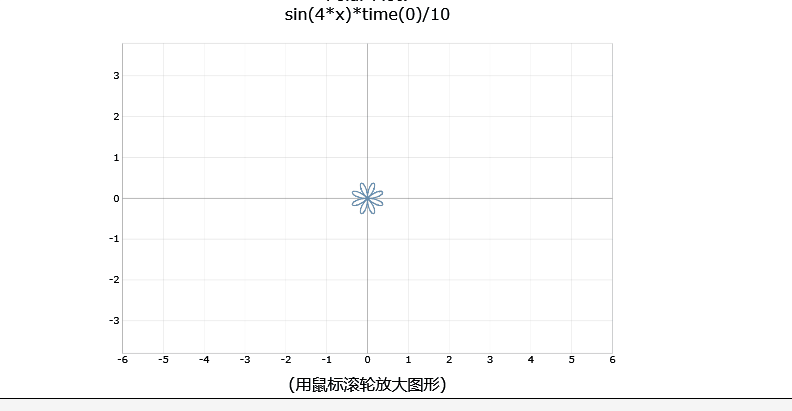flowering by polarplot( sin(4*x)*timer(6) )
65.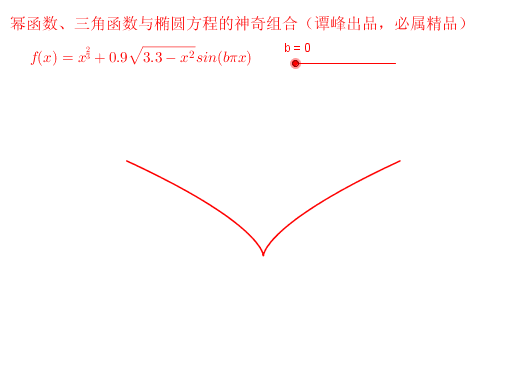I love you by my heart. abs(x)^(2/3)+0.9*sqrt(3.3-x^2)*sin(pi*x*timer(30))
66.A plane curve which is progressively drawn. (Cycloid)
67.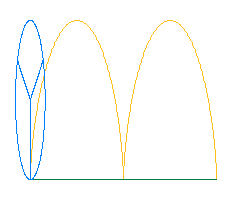The Cycloid is the orbit of a point on a circle rolling along a straight line. Here you can see how this is done precisely, with multiple curve plotting.
68.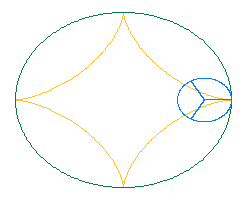By the same principle, the astroid (cos^3(t),sin^3(t)) is the orbit of a point on a circle of radius 1/4 rolling inside a fixed circle of radius 1.
69.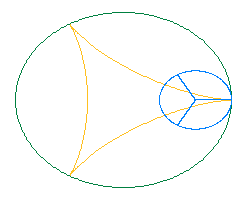We have also the astroid with 3 arcs.
70.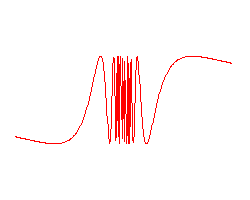Zooming effect on the curve sin(1/x).
71.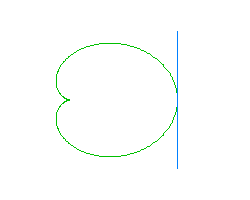A moving tangent along a plane parametric curve (cardioid).
72.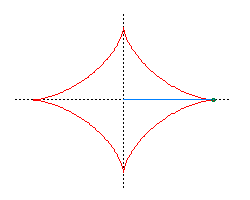The moving tangent along the astroid has a special property: the segment of the tangent cut out by the coordinate axes is of constant length.
73.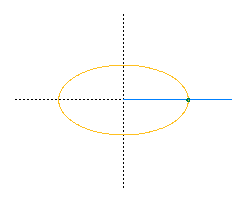How an ellipse is drawn by a point on a segment of fixed length with two extremities on the x and y axes respectively.
74.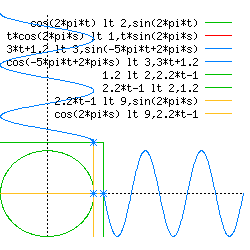An animation which illustrates the functions sin and cos as vertical and horizontal positions of a moving point on a circle.
75.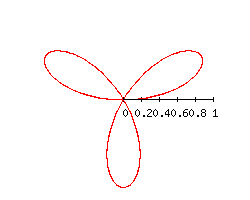Rotating and growing trefoil.
76. Cornu Spiral - the Fresnel cosine and sine integrals.
77. choreographies
78. coloring butterfly
79. coloring square
80. Unwrapping Circle
81. Weierstrass

• example 例题
• graph 制图
• graphics 制图法
• drawing 画画

• Paul Masson
• Xiao Gang